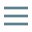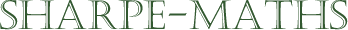Previous topic:
Multiples & factors
Current topic:
Primes
Next topic:
HCF (GCD) & LCM

### Prime numbers

Prime numbers are the basic building blocks that make up all other whole numbers. Some numbers fall apart in short order: 12 is an examples. 12 has 2 different ways of breaking down, $2 \times 6, 3 \times 4$. A useful metaphor for the different types of numbers is the make up of chemical molecules. Some molecules are highly reactive like hydrogen and potassium, others much less so, like helium or gold. Reactive numbers, which we call composite numbers, are those with a bunch of different factors, and non reactive numbers, which we call prime numbers don't break down at all.

A prime number has exactly two distinct (different) factors. In other words, primes cannot be expressed as the product (result of multiplying) of other numbers, whereas composite numbers can be expressed as a product of other prime numbers.

This is called the Fundamental Theorem of Arithmetic which is, as its name implies, at the core of multiplication processes and many other parts of arithmetic or Number Theory.

As an example, take the numbers 36 and 37. They occur right next door to each other but couldn't be more different in their underlying structure.

\begin{aligned} 36 &= 1 \times 36\\&=2 \times 18\\&=3 \times 12\\&=4 \times 9\\&=6 \times 6 \end{aligned}

$36$ has $9$ factors (the $6$ is repeated here because it is a square number. This explains why only square numbers have an odd number of factors.

Now $37$, on the other hand can't be broken down at all. The only way $37$ can be written as a product of whole numbers is:

$37 = 1 \times 37$

The science fiction writer, Harry Harrison (author of the Stainless Steel Rat books and many others) called them "Crunchy numbers" in a novel he wrote with the AI scientist, Marvin Minsky. I have always liked that image. Prime numbers are hard, stolid and stable. Composite numbers, on the other hand are squelchy, unstable things which fall apart at the slightest opportunity.

Primes have fascinated mathematicians and philosophers for many millennia and with the passage of time, they have become more inscrutable, unfathomable and difficult. When I was studying for my degree back in the 1970s, the study of prime numbers was a rather arcane, rarefied and useless area of study: the purest of Pure Mathematics. Nowadays, we use prime numbers (without knowing it) every time you make an online purchase, or access an SSL (secure) website.

The (probably) most famous unsolved problem in mathematics is called "The Riemann Hypothesis" and it concerns, indirectly, the sequence of the prime numbers. It was first suggested by the brilliant German mathematician, Bernhard Riemann in 1859 and it has puzzled and fascinated mathematicians ever since. It is not for the faint hearted and should only be researched by those with fairly high levels of mathematical knowledge. It is the Chomolungma (Mt Everest, if you insist) of mathematics.